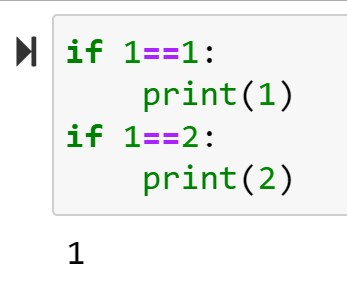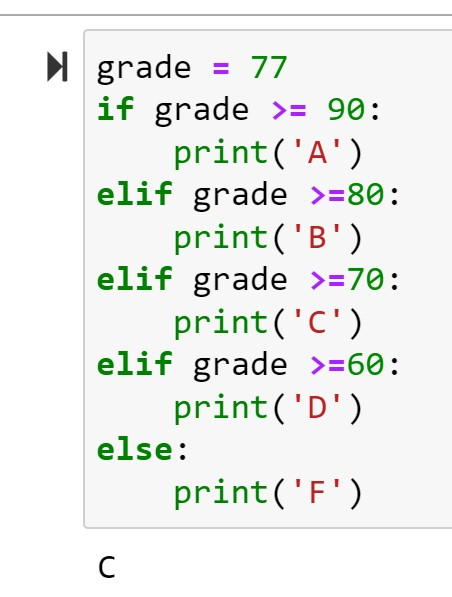# Python: Conditional Logic

Conditional logic is the decision making arm of programming. With conditional logic, we examine a condition and instruct the computer what to do based on the condition. If you have had any exposure to computer programming, the IF THEN statement should immediately come to mind here.

## Comparison Operators

Before we can start learning condition logic, we need to take a look at the comparison operators found in Python

• == : Is equal to – 1 == 1
• != : Is not equal to – 1 != 2
• <> : Is not equal to – 1 <> 2
• > : Greater than – 2 > 1
• < : Less than – 1 < 2
• >= : Greater then or equal to – 2>=1 and 2>=2
• <=: Less then or equal to – 1<=2 and 1<=1

Comparison operators return a boolean condition (True or False) – **note that in Python True and False are capitalized words.

So 1==1 returns True

1==2 returns False

## If Statement

Python’s basic conditional logic statement is the IF statement. It works the same as the IF THEN statements you may have learned in other languages, but Python takes advantage of its use of whitespace, negating the need for the keyword THEN.

### Syntax:

if CONDITION:
Notice in both examples below, only the condition that returns True is executedThe IF statement can also compare strings:Try your code in our online Python console:## Else:

In this next example, I am tackling two topics.

1. You can use variables in an IF statement. They work exactly the same as if we were using numbers
2. ELSE: In a simple IF only statement, if the condition is False, nothing happens. But what if you want something to happen? Add an ELSE to the mix.

The way it works is simple. IF condition True, do this

ELSE (if not True), do this instead.## Nested IF ELSE:

Most real world scenarios are  more complex than a simple Yes or No.

To model more complex scenarios in computer code, we need to use nested IF ELSE. In the example below, we have 3 possible outcomes, if 18 and over, you are an Adult, if 13 to 17, you are a teenage, and if you are under 13, you are a child.

The example works through that by placing an IF ELSE statement inside another IF ELSE statement. The result of the first ELSE is to check for another condition.

Notice the use of whitespace here. You can see that entirety of the second IF ELSE statement belongs under the first ELSE by the way the code is indented.Try your code in our online Python console:## ELIF:

It is not hard to picture how unruly a nested IF ELSE statement could get if you had say 5 conditions you wanted to test against. Luckily Python provides a more elegant solution, the ELIF:

See in the example below how the ELIF functions as a combination ELSE IF statement. It cuts out an entire line of code for each condition and lets the code remain neatly lined up. I personally find this easier to read as well. The more complex your code becomes, the more you will appreciate readability.## Bonus: Using IF inside a For Loop

Just for fun, look at the example below:

Check out the output. Is that what you expected? Remember range(0,10,2) returns the numbers from 0 to 10 (not including 10) counting by 2’s. [0,2,4,6,8].  Putting an if statement inside the loop, allows you to evaluated each value in the iteration. When i equals 6, the if statement tells Python to print the word.

If you enjoyed this lesson, click LIKE below, or even better, leave me a COMMENT

Last Lesson: Tuples and Sets

Next Lesson: Loops

Back to Python Course: Course